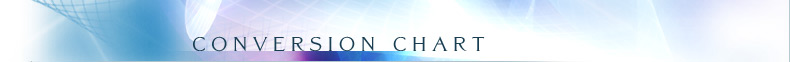# Units Conversion Chart

NOVA uses the U.S. customary system of weights and measures. If you would like to know the metric equivalent, please consult the conversion chart below. The chart is reprinted with permission from The Associated Press Stylebook and Libel Manual (Addison Wesley, 1998), our official stylebook.Into Metric Out of Metric If youknow Multiplyby ToGet If youknow Multiplyby ToGet Length Length inches 2.54 centimeters millimeters 0.04 inches foot 30 centimeters centimeters 0.4 inches yards 0.91 meters meters 3.3 feet miles 1.6 kilometers kilometers 0.62 miles Area Area sq. inches 6.5 sq. centimeters sq. centimeters 0.16 sq. inches sq. feet 0.09 sq. meters sq. meters 1.2 sq. yards sq. yards 0.8 sq. meters sq. kilometers 0.4 sq. miles sq. miles 2.6 sq. kilometers hectares 2.47 acres acres 0.4 hectares Mass (Weight) Mass (Weight) ounces 28 grams grams 0.035 ounces pounds 0.45 kilograms kilograms 2.2 pounds short ton 0.9 metric ton metric tons 1.1 short tons Volume Volume teaspoons 5 milliliters milliliters 0.03 fluid ounces tablespoons 15 milliliters liters 2.1 pints fluid ounces 30 milliliters liters 1.06 quarts cups 0.24 liters liters 0.26 gallons pints 0.47 liters cubic meters 35 cubic feet quarts 0.95 liters cubic meters 1.3 cubic yards gallons 3.8 liters cubic feet 0.03 cubic meters cubic yards 0.76 cubic meters Temperature Temperature Fahrenheit Subtract 32, then multiply by 5/9ths Celsius Celsius Multiply by 9/5ths, then add 32 Fahrenheit